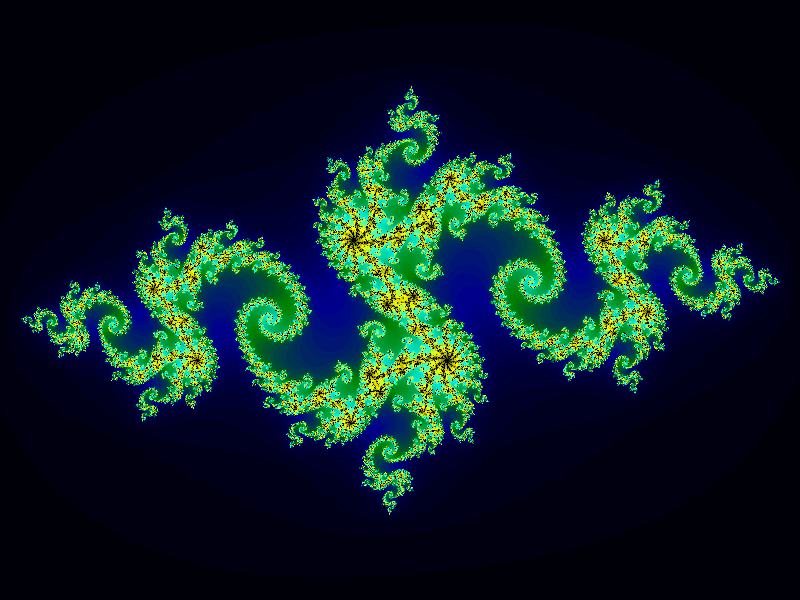# Julia fractal in generativepy

Martin McBride
2022-01-22

The Julia set is a close relative of the Mandelbrot set. There are far more variants of the Julia set, in fact every point in the Mandelbrot set corresponds to a complete Julia set.

## Basic equation

The equation for the Julia set is:

znext = z*z + C


Where z is a complex variable, and C is a complex number constant. Just like the Mandelbrot case, we can rewrite this equation as a pair of equations in x and y:

xnext = x*x - y*y + C1
ynext = 2*x*y + C2


## Plotting the fractal

We start by choosing values for C1 and C2. This value will remain constant for the entire fractal. We can choose any value we want for C1 and C2, and different pairs will often give a different result (although not every pair will produce an interesting result).

To create the fractal we loop over every possible combination of (x0, y0) values (each corresponding to a pixel in the final image). For each pair of values:

• We use (x, y) as the initial values in the equations above.
• We loop over the equations, using the current x and y to create the next x and y. The loop runs until either:
• The distance of point (x, y) from the origin is greater than 2 or
• We have looped more that 256 times.

In the first case, we say that the initial point diverges, so the point is not part of the Julia set. We record the number of iterations that it takes to diverge (ie how many iteration until the distance is greater than 2) and use that to select a colour for the pixel.

In the second case, we say that the initial point converges, so the point is part of the Julia set. The pixel will be coloured black.

## Colorising the values

In a different article we saw how to colour a Tinkerbell fractal. We will use a similar technique here.

In summary:

• We will use make_nparray_data to write the count values for each pixel to an integer NumPy array dimensions height x width x 1.
• Use a colorise function to convert the counts array to a height x width x 3 array (an RGB value for each pixel).
• Use save_nparray_image to save the RGB data as an image.

Here is the result:## The complete code

Here is the complete code for the Julia set:

from generativepy.bitmap import Scaler
from generativepy.nparray import make_nparray_data, save_nparray, load_nparray, make_npcolormap, apply_npcolormap, save_nparray_image
from generativepy.color import Color
from generativepy.analytics import print_stats, print_histogram
import numpy as np

MAX_COUNT = 256
C1 = -0.79
C2 = 0.15

def calc(x, y):
for i in range(MAX_COUNT):
x, y = x*x - y*y + C1, 2*x*y + C2
if x*x + y*y > 4:
return i+1
return 0

def paint(image, pixel_width, pixel_height, frame_no, frame_count):
scaler = Scaler(pixel_width, pixel_height, width=3.2, startx=-1.6, starty=-1.2)

for px in range(pixel_width):
for py in range(pixel_height):
x, y = scaler.device_to_user(px, py)
count = calc(x, y)
image[py, px] = count

def colorise(counts):
counts = np.reshape(counts, (counts.shape, counts.shape))

colormap = make_npcolormap(MAX_COUNT+1,
[Color('black'), Color('darkblue'), Color('green'), Color('cyan'), Color('yellow'), Color('black')],
[16, 16, 32, 32, 128])

outarray = np.zeros((counts.shape, counts.shape, 3), dtype=np.uint8)
apply_npcolormap(outarray, counts, colormap)
return outarray

data = make_nparray_data(paint, 800, 600, channels=1)

frame = colorise(data)


This code is available on github in blog/fractals/julia.py.

Here is what the colorise function does:

• Reshape our counts array from (height, width, 1) to (height, width).
• Create a colormap with MAX_COUNT+1 elements.
• Create an output array that is height by width by 3, to hold RGB image data. The array is of type uint8, which is an unsigned byte value. We call apply_npcolormap to convert the normalised count array into an RGB image array.

The colormap goes from black to dark blue to green to cyan to white. However, we have also supplied a bands array [16, 16, 32, 32, 128]. This specifies the relative size of each band. This means that first three bands are quite small, but the cyan-yellow band is bigger, and the yellow-white band is even bigger. This equalises the transitions over the image. so that the less interesting areas well away from the fractal boundary have subtle colour changes, whereas the more interesting part of the image is enhanced by a rapid green-yellow-cyan-white change.

You can easily experiment with other colour schemes.

## Comparison with Mandelbrot

If you looked at the Mandelbrot fractal, you will probably have noticed that they basically use the same equation:

xnext = x*x - y*y + C1
ynext = 2*x*y + C2


The difference is:

• For the Mandelbrot set, we try every possible combination of c1 and c2. We always start with initial values of zero for x and y. The pixel (c1, c2) is set according to the divergence time.
• For the Julia set, we used a fixed value of C1 and C2. We we try every possible combination of initial values for x and y. The pixel (x, y) is set according to the divergence time.

## Variants

Different combinations of C1 and C2 will create a different fractal. However, many of the fractals will not be interesting, for example they might be completely black.

Generally the most interesting combination occur for values of (C1, C2) that are just outside the boundary of the Mandelbrot set.

## Sign up to the Creative Coding Newletter

Join my newsletter to receive occasional emails when new content is added, using the form below:

## Popular tags

ebooks fractal generative art generativepy generativepy tutorials github koch curve l systems mandelbrot open source productivity pysound python recursion scipy sine sound spriograph tinkerbell turtle writing